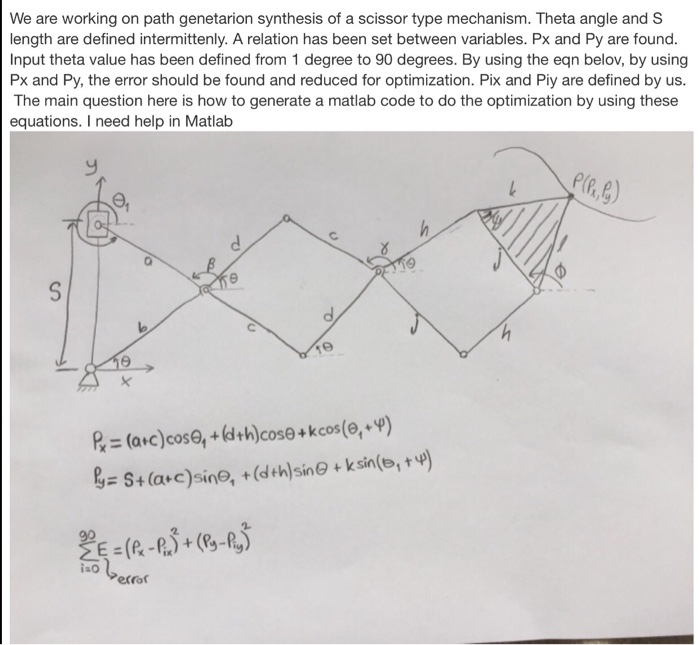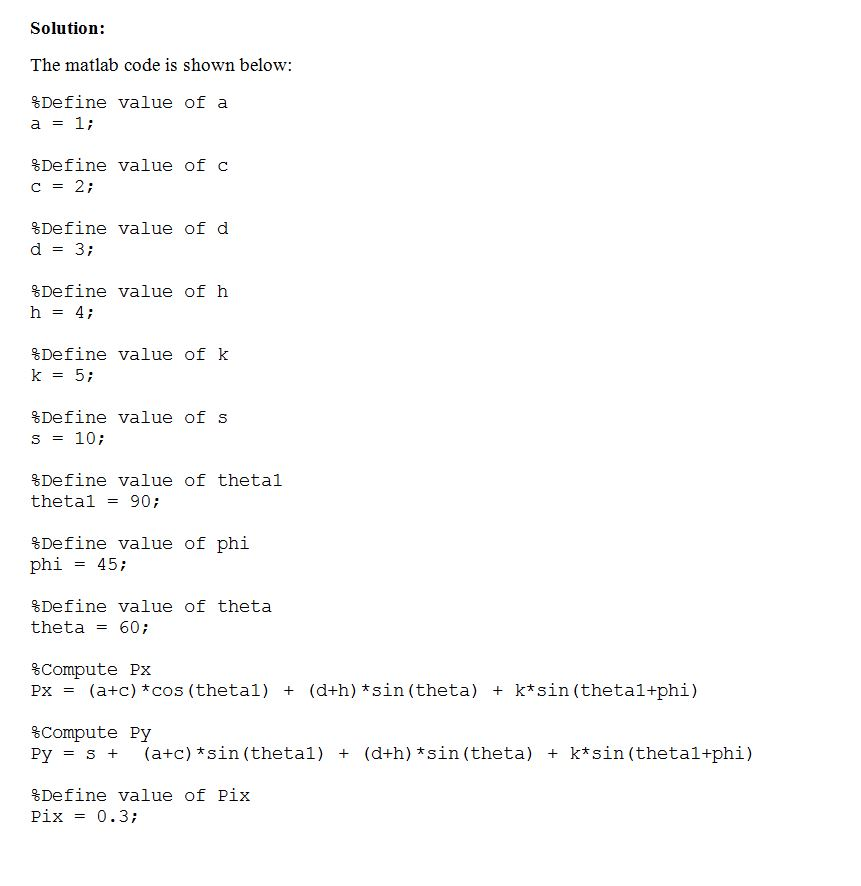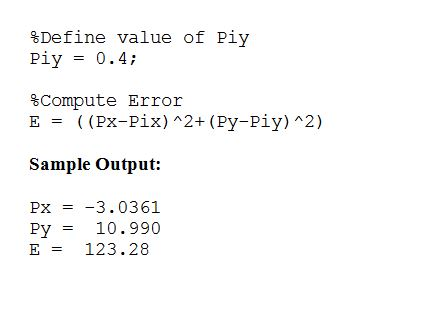# Question & Answer: We are working on path genetarion synthesis of a scissor type mechanism. Theta angle and S length are…..

We are working on path genetarion synthesis of a scissor type mechanism. Theta angle and S length are defined intermittenly. A relation has been set between variables. Px and Py are found. Input theta value has been defined from 1 degree to 90 degrees. By using the eqn belov, by using Px and Py, the error should be found and reduced for optimization. Pix and Piy are defined by us. The main question here is how to generate a matlab code to do the optimization by using these equations. I need help in Matlab P_x = (a+c)cos theta_1 + (d + h)cos theta + k cos(theta_1 + psi) P_y = S + (a + c)sin theta_1 + (d + h)sin theta + k sin(theta_1 + psi) sigma^90_i=0 E = (P_x – P_ix)^2 + (P_y + P_iy)^2Code:

Don't use plagiarized sources. Get Your Custom Essay on
Question & Answer: We are working on path genetarion synthesis of a scissor type mechanism. Theta angle and S length are…..
GET AN ESSAY WRITTEN FOR YOU FROM AS LOW AS \$13/PAGE

%Define value of a

a = 1;

%Define value of c

c = 2;

%Define value of d

d = 3;

%Define value of h

h = 4;

%Define value of k

k = 5;

%Define value of s

s = 10;

%Define value of theta1

theta1 = 90;

%Define value of phi

phi = 45;

%Define value of theta

theta = 60;

%Compute Px

Px = (a+c)*cos(theta1) + (d+h)*sin(theta) + k*sin(theta1+phi)

%Compute Py

Py = s + (a+c)*sin(theta1) + (d+h)*sin(theta) + k*sin(theta1+phi)

%Define value of Pix

Pix = 0.3;

%Define value of Piy

Piy = 0.4;

%Compute Error

E = ((Px-Pix)^2+(Py-Piy)^2)